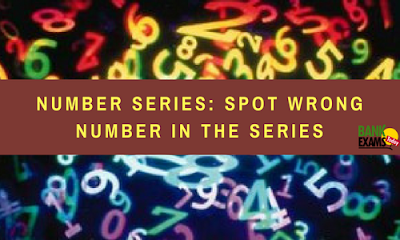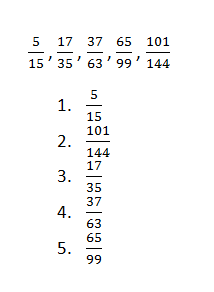# Number Series: Spot Wrong Number in the SeriesDirections (1-5): In the following number series one number is wrong. Find out the wrong number.

Ques 1.
11, 28, 79, 231, 691, 2068
1. 79
2. 28
3. 691
4. 231
5. 2068

Ques 2.
141, 157, 120, 185, 85, 229
1. 120
2. 85
3. 157
4. 185
5. 229

Ques 3.
168, 195, 224, 255, 289, 323
1. 168
2. 224
3. 289
4. 323
5. 255

Ques 4.Ques 5.
34, 85, 153, 187, 214, 238
1. 187
2. 214
3. 34
4. 153
5. 85

1. 4;
The series is:
11 x 3 - 5 = 28
28 x 3 - 5 = 79
79 x 3 - 5 = 232 ≠ 231
232 x 3 - 5 = 691
691 x 3 - 5 = 2068
Hence, there should be 232 in place of 231

2. 1;
The series is :
141 + 4² = 157
157 - 6² = 121 ≠ 120
121 + 8² = 185
185 - 10² = 85
85 + 12² = 229
Hence, there should be 121 in place of 120

3. 3;
The series is :
13² - 1 = 168, 14² - 1 = 195, 15² - 1 = 224
16² - 1 = 255 , 17² - 1 = 288 ≠ 289, 18² - 1 = 323
Hence, there should be 288 in place of 289

4. 2;
The series is:5. 2;
All the numbers in the series are multiple of 17 except 2014.#### What's trending in BankExamsToday

Smart Prep Kit for Banking Exams by Ramandeep Singh - Download here